# Texas Go Math Grade 5 Lesson 3.8 Answer Key Zeros in the Product

Refer to our Texas Go Math Grade 5 Answer Key Pdf to score good marks in the exams. Test yourself by practicing the problems from Texas Go Math Grade 5 Lesson 3.8 Answer Key Zeros in the Product.

## Texas Go Math Grade 5 Lesson 3.8 Answer Key Zeros in the Product

Unlock the Problem

Connect When decimals are multiplied, the product may not
have enough digits to place the decimal point. In these cases,
you may need to write additional zeros.

Students are racing garden snails and measuring the
distance the snails travel in 1 minute.
Chris’s snail travels a distance of 0.2 foot. Jamie’s snail
travels 0.4 times as far as Chris’s snail. How far does Jamie’s snail travel?

Using the given information, describe what you are being asked to find.
Jamie’s snail travel 0.08 foot,

Multiply. 0.4 × 0.2

Explanation:
STEP 1:
Multiply as with whole numbers.

STEP 2:
Determine the position of the decimal point in the product.
Since tenths are being multiplied by tenths, the
product will show ____0.08______.

STEP 3:
Place the decimal point.
Are there enough digits in the product to place the decimal point? _____Yes_____
Write zeros, as needed, to the left of the whole number product to place the decimal point.
So, Jamie’s snail travels a distance of ____0.08______ foot.0.4 x 0.2 = 0.08 foot,

Explanation:
STEP 1:
Multiply as with whole numbers,

STEP 2:
Determining the position of the decimal point in the product.
Since tenths are being multiplied by tenths, the product will show 0.08,

STEP 3:
Placing the decimal point, Yes there are enough digits in the product in
placing the decimal point, Writing zeros, as needed, to the left of the
whole number product to place the decimal point.
So, Jamie’s snail travels a distance of  0.08 foot.

Math Talk
Mathematical Processes

Explain how you know when to write zeros in the product place a decimal point.
Multiplying as with whole numbers,
Determining the position of the decimal point in the product,
Placing the decimal point. Writing zeros to the left of the whole number product as needed.

Example Multiply money.

Explanation:
Multiplying  0.2 × $0.30, STEP 1: Multiply as with whole numbers. Think: The factors are 30 hundredths and 2 tenths. What are the whole numbers you will multiply? STEP 2: Determine the position of the decimal point in the product. Since hundredths are being multiplied by tenths, the product will show ____600_________. STEP 3: Place the decimal point. Write zeros to the left of the whole number product as needed. Since the problem involves dollars and cents, what place value should you use to show cents?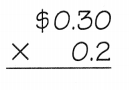So, 0.2 ×$0.30 is ____0.06 cents_______.
0.2 X $0.30 = 0.06 cents, Explanation: STEP 1: Multiplying as with whole numbers. Thinking: The factors are 30 hundredths and 2 tenths. We multiply the whole numbers, STEP 2: Determining the position of the decimal point in the product. Since tenths are being multiplied by tenths, the product will show 600, STEP 3: Placing the decimal point. Writing zeros to the left of the whole number product as needed. Since the problem involves dollars and cents, the place value we should use to show cent is 0.06. Share and Show Writes zeros in the product. Question 1.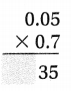Think: Hundredths are multiplied by tenths. What should be the place value of the product? Answer: 0.05 X0.7 0.035 The place value of the product is 0.035, Explanation: STEP 1: Multiplying as with whole numbers. Thinking: The factors are 5 hundredths and 7 tenths. We multiply the whole numbers, STEP 2: Determining the position of the decimal point in the product. Since hundredths are being multiplied by tenths, the product will show 35, STEP 3: Placing the decimal point. Writing zeros to the left of the whole number product as needed. The place value of the product is 0.035. Question 2.Answer: 0.2 X0.3 0.06 The place value of the product is 0.06, Explanation: STEP 1: Multiplying as with whole numbers. Thinking: The factors are 2 tenths and 3 tenths. We multiply the whole numbers, STEP 2: Determining the position of the decimal point in the product. Since tenths are being tenths by tenths, the product will show 6, STEP 3: Placing the decimal point. Writing zeros to the left of the whole number product as needed. The place value of the product is 0.06. Question 3.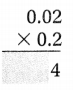Answer: 0.02 X0.2 0.004 The place value of the product is 0.004, Explanation: STEP 1: Multiplying as with whole numbers. Thinking: The factors are 2 hundredths and 2 tenths. We multiply the whole numbers, STEP 2: Determining the position of the decimal point in the product. Since hundredths are being multiplied by tenths, the product will show 4, STEP 3: Placing the decimal point. Writing zeros to the left of the whole number product as needed. The place value of the product is 0.004. Find the product. Question 4.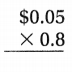Answer:$0.05
X0.8
0.040
The place value of the product  is 0.040,

Explanation:
STEP 1:
Multiplying as with whole numbers.
Thinking: The factors are 5 hundredths and 8 tenths.
We multiply the whole numbers,

STEP 2:
Determining the position of the decimal point in the product.
Since hundredths are being multiplied by tenths,
the product will show 40,

STEP 3:
Placing the decimal point. Writing zeros to the left of the
whole number product as needed.
The place value of the product  is 0.040.

Question 5.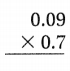0.09
X0.7
0.063
The place value of the product  is 0.063,

Explanation:
STEP 1:
Multiplying as with whole numbers.
Thinking: The factors are 9 hundredths and 7 tenths.
We multiply the whole numbers,

STEP 2:
Determining the position of the decimal point in the product.
Since hundredths are being multiplied by tenths,
the product will show 63,

STEP 3:
Placing the decimal point. Writing zeros to the left of the
whole number product as needed.
The place value of the product  is 0.063.

Question 6.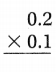0.2
X0.1
0.02
The place value of the product  is 0.02,

Explanation:
STEP 1:
Multiplying as with whole numbers.
Thinking: The factors are 2 tenths and 7 tenths.
We multiply the whole numbers,

STEP 2:
Determining the position of the decimal point in the product.
Since tenths are being multiplied by tenths,
the product will show 2,

STEP 3:
Placing the decimal point. Writing zeros to the left of the
whole number product as needed.
The place value of the product  is 0.02.

Math Talk
Mathematical Processes

Explain why 0.04 × 0.2 has the same product as 0.4 × 0.02.
Both the product results are the same 0.008,

Explanation:
If we multiply 0.04 X 0.2 we get
0.04
X0.2
0.008
and if we multiply 0.4 X 0.02 we get
0.4
X0.02
0.008
therefore 0.04 × 0.2 has the same product as 0.4 × 0.02.

Unlock the Problem

Question 7.
H.O.T. Multi-Step On an average day, a garden snail can travel about 0.05 mile.
The snail travels 0.2 times as far as the average distance on Day 1.
It travels 0.6 times as far as the average distance on Day 2.
How far does it travel in two days?
(A) 0.01 mile
(B) 0.03 mile
(C) 0.1 mile
(D) 0.04 mile

a. What are you being asked to find?
Snail travels far in two days is (D) 0.04 mile,

Explanation:
Given on an average day, a garden snail can travel about 0.05 mile.
The snail travels 0.2 times as far as the average distance on Day 1.
It travels 0.6 times as far as the average distance on Day 2.
So snail travels far in two days is on day 1- 0.05 mile X 0.2 = 0.01 mile and
on day 2 – 0.05 mile X 0.6 = 0.03 mile in total 0.01mile + 0.03 mile = 0.04 mile
matches with (D).

b. What information will you use to solve the problem?
How much snail travels,

Explanation:
By using given information of distance traveled by the snail on
average day, day 1 and day 2.

c. Which operations can you use to solve the problem?

Explanation:
Multiplication for calculating distance traveled by the snail with
average day and  day 1, day 2.
Addition for total distance traveled by the snail on  day 1, day 2.

d. Show how you will solve the problem.
By solving the problem snail travels far in two days is 0.04 mile,

Explanation:
Solving the given on an average day, a garden snail can travel about 0.05 mile.
The snail travels 0.2 times as far as the average distance on Day 1.
It travels 0.6 times as far as the average distance on Day 2.
So snail travels far in two days is on day 1- 0.05 mile X 0.2 = 0.01 mile and
on day 2 – 0.05 mile X 0.6 = 0.03 mile in total 0.01mile + 0.03 mile = 0.04 mile.

e. Fill in the bubble for the correct answer choice above.
(D) 0.04 mile,

Explanation:
The bubble for the correct answer choice above is (D) 0.04 mile.

Question 8.
H.O.T. Michael multiplies 0.2 by a number. He records the product as 0.008.
What number did Michael use?Michael uses 0.04,

Explanation:
Given Michael multiplies 0.2 by a number. He records the product as 0.008.
Therefore the number did Michael used is 0.008 ÷ 0.2 = 0.04.

Question 9.
Apply Newaye is making a salt solution. The instructions ask for
0.07 kilograms of salt to be added to every liter of water.
How much salt should he use if he wants to make a solution with 0.5 liter of water?
(A) 0.35 kilogram
(B) 0.035 kilogram
(C) 0.305 kilogram
(D) 3.5 kilograms
(B) 0.035 kilogram,

Explanation:
Applying Newaye is making a salt solution. The instructions ask for
0.07 kilograms of salt to be added to every liter of water salt should he use
if he wants to make a solution with 0.5 liter of water is
0.07 kilograms X 0.5 liter = 0.035 kilogram matches with (B).

Question 10.
Al buys raw peanuts for $0.40 for a pound. He roasts them and sells them in 0.2-pound bags. How much does a bag of peanuts cost Al? (A)$8.00
(B) $0.80 (C)$0.08
(D) $0,008 Answer: (C)$0.08,

Explanation:
Given AI buys raw peanuts for $0.40 for a pound. He roasts them and sells them in 0.2-pound bags. Bag of peanuts does AI cost is$0.40 X 0.2 = $0.08 which matches with (C). Question 11. Multi-Step Al finds a new supplier. He can buy raw peanuts for$0.30 for a pound. If he sells the peanuts in 0.2-pound bags for $2.50 a bag, how much profit is made on each bag? (A)$2.44
(B) $2.04 (C)$1.90
(D) $0.60 Answer: (A)$2.44,

Explanation:
Given Al finds a new supplier. He can buy raw peanuts for $0.30 for a pound. If he sells the peanuts in 0.2-pound bags for$2.50 a bag,
So he bought 0.2 X $0.30 =$0.06,So profit he made on each bag is
$2.50 –$0.06 = $2.44 which matches with (A). Texas Test Prep Question 12. Apply In a science experiment, Tania uses 0.8 ounce of water to create a reaction. She wants the next reaction to be 0.1 times the size of the previous reaction. How much water should she use? (A) 0.08 ounce (B) 0.9 ounce (C) 0.8 ounce (D) 0.09 ounce Answer: (A) 0.08 ounce, Explanation: Applying in a science experiment, Tania uses 0.8 ounce of water to create a reaction. She wants the next reaction to be 0.1 times the size of the previous reaction. So amount of water should she use is 0.8 X 0.1 = 0.08 ounce which matches (A). ### Texas Go Math Grade 5 Lesson 3.8 Homework and Practice Answer Key Write zeros in the product. Question 1.Answer: 0.06 X0.4 0.024 The place value of the product is 0.024, Explanation: STEP 1: Multiplying as with whole numbers. Thinking: The factors are 6 hundredths and 4 tenths. We multiply the whole numbers, STEP 2: Determining the position of the decimal point in the product. Since hundredths are being multiplied by tenths, the product will show 35, STEP 3: Placing the decimal point. Writing zeros to the left of the whole number product as needed. The place value of the product is 0.024. Question 2.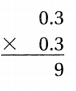Answer: 0.3 X0.3 0.09 The place value of the product is 0.09, Explanation: STEP 1: Multiplying as with whole numbers. Thinking: The factors are 9 tenths and 9 tenths. We multiply the whole numbers, STEP 2: Determining the position of the decimal point in the product. Since tenths are being tenths by tenths, the product will show 9, STEP 3: Placing the decimal point. Writing zeros to the left of the whole number product as needed. The place value of the product is 0.09. Question 3.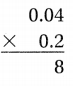Answer: 0.04 X0.2 0.008 The place value of the product is 0.008, Explanation: STEP 1: Multiplying as with whole numbers. Thinking: The factors are 4 hundredths and 2 tenths. We multiply the whole numbers, STEP 2: Determining the position of the decimal point in the product. Since hundredths are being multiplied by tenths, the product will show 8, STEP 3: Placing the decimal point. Writing zeros to the left of the whole number product as needed. The place value of the product is 0.008. Question 4.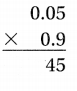Answer: 0.05 X0.9 0.045 The place value of the product is 0.045, Explanation: STEP 1: Multiplying as with whole numbers. Thinking: The factors are 5 hundredths and 9 tenths. We multiply the whole numbers, STEP 2: Determining the position of the decimal point in the product. Since hundredths are being multiplied by tenths, the product will show 45, STEP 3: Placing the decimal point. Writing zeros to the left of the whole number product as needed. The place value of the product is 0.045. Question 5.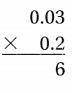Answer: 0.03 X0.2 0.006 The place value of the product is 0.006, Explanation: STEP 1: Multiplying as with whole numbers. Thinking: The factors are 3 hundredths and 2 tenths. We multiply the whole numbers, STEP 2: Determining the position of the decimal point in the product. Since hundredths are being multiplied by tenths, the product will show 6, STEP 3: Placing the decimal point. Writing zeros to the left of the whole number product as needed. The place value of the product is 0.006. Question 6.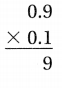Answer: 0.9 X0.1 0.09 The place value of the product is 0.09, Explanation: STEP 1: Multiplying as with whole numbers. Thinking: The factors are 9 tenths and 1 tenths. We multiply the whole numbers, STEP 2: Determining the position of the decimal point in the product. Since tenths are being multiplied by tenths, the product will show 9, STEP 3: Placing the decimal point. Writing zeros to the left of the whole number product as needed. The place value of the product is 0.09. Find the product. Question 7.Answer:$0.04
X0.5
0.02
The place value of the product  is 0.02,

Explanation:
STEP 1:
Multiplying as with whole numbers.
Thinking: The factors are 4 hundredths and 5 tenths.
We multiply the whole numbers,

STEP 2:
Determining the position of the decimal point in the product.
Since hundredths are being multiplied by tenths,
the product will show 20,

STEP 3:
Placing the decimal point. Writing zeros to the left of the
whole number product as needed.
The place value of the product  is 0.02.

Question 8.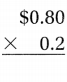$0.80 X0.2 0.16 The place value of the product is$0.16,

Explanation:
STEP 1:
Multiplying as with whole numbers.
Thinking: The factors are 8 tenths and 2 tenths.
We multiply the whole numbers,

STEP 2:
Determining the position of the decimal point in the product.
Since tenths are being multiplied by tenths,
the product will show 16,

STEP 3:
Placing the decimal point. Writing zeros to the left of the
whole number product as needed.
The place value of the product  is $0.16. Question 9.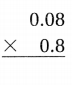Answer:$0.08
X0.8
0.064
The place value of the product  is 0.064,

Explanation:
STEP 1:
Multiplying as with whole numbers.
Thinking: The factors are 8 hundredths and 8 tenths.
We multiply the whole numbers,

STEP 2:
Determining the position of the decimal point in the product.
Since hundredths are being multiplied by tenths,
the product will show 64,

STEP 3:
Placing the decimal point. Writing zeros to the left of the
whole number product as needed.
The place value of the product  is 0.02.

Question 10.$0.02 X0.3 0.006 The place value of the product is 0.006, Explanation: STEP 1: Multiplying as with whole numbers. Thinking: The factors are 2 hundredths and 3 tenths. We multiply the whole numbers, STEP 2: Determining the position of the decimal point in the product. Since hundredths are being multiplied by tenths, the product will show 6, STEP 3: Placing the decimal point. Writing zeros to the left of the whole number product as needed. The place value of the product is 0.006. Question 11.Answer: 0.1 X0.7 0.07 The place value of the product is 0.07, Explanation: STEP 1: Multiplying as with whole numbers. Thinking: The factors are 1 tenths and 7 tenths. We multiply the whole numbers, STEP 2: Determining the position of the decimal point in the product. Since tenths are being multiplied by tenths, the product will show 7, STEP 3: Placing the decimal point. Writing zeros to the left of the whole number product as needed. The place value of the product is 0.07. Question 12.Answer:$0.60
X0.5
0.30
The place value of the product  is 0.30,

Explanation:
STEP 1:
Multiplying as with whole numbers.
Thinking: The factors are 6 tenths and 5 tenths.
We multiply the whole numbers,

STEP 2:
Determining the position of the decimal point in the product.
Since tenths are being multiplied by tenths,
the product will show 3,

STEP 3:
Placing the decimal point. Writing zeros to the left of the
whole number product as needed.
The place value of the product  is $0.30. Problem Solving Question 13. The distance from Mario’s house to the park is 0.5 mile. His friend Kirk’s house is 0.3 times as far from Mario’s house as Mario’s house is from the park. What is the distance from Mario’s house to Kirk’s house? Answer: The distance from Mario’s house to Kirk’s house is 0.5 mile X 0.3 = 0.15 miles, Explanation: Given the distance from Mario’s house to the park is 0.5 mile. His friend Kirk’s house is 0.3 times as far from Mario’s house as Mario’s house is from the park. So the distance from Mario’s house to Kirk’s house is 0.5 mile X 0.3 = 0.15 miles. Question 14. A scientist determines the mass of two specimens. Specimen A has a mass of 0.08 gram. Specimen B has a mass 0.9 times the mass of Specimen B. What is the mass of Specimen B? Answer: 0.9 times the mass of Specimen B, Explanation: Given A scientist determines the mass of two specimens. Specimen A has a mass of 0.08 gram. Specimen B has a mass 0.9 times the mass of Specimen B. So the mass of Specimen B is 0.9 times the mass of Specimen B. Lesson Check Fill in the bubble completely to show your answer. Question 15. Joel makes a card for his dad. The length of the card is 0.7 foot. The width of the card is 0.4 times the length. What is the width of the card? (A) 0.11 foot (B) 0.74 foot (C) 0.28 foot (D) 0.21 foot Answer: (C) 0.28 foot, Explanation: Given Joel makes a card for his dad. The length of the card is 0.7 foot. The width of the card is 0.4 times the length. Therefore the width of the card is 0.7 foot X 0.4 = 0.28 foot. Question 16. Carlos uses a ruler to measure the distances between cities on his map of the U.S. The first distance measures 0.6 meter. The second distance he measures is 0.1 times the first measurement. What is the second measurement? (A) 0.6 meter (B) 0.06 meter (C) 6 meters (D) 0.006 meter Answer: (B) 0.06 meter, Explanation: Given Carlos uses a ruler to measure the distances between cities on his map of the U.S. The first distance measures 0.6 meter. The second distance Carlos measures is 0.1 times the first measurement. So the second measurement is 0.6 X 0.1 =0.06 meter, matches with (B). Question 17. The product is 0.016. One factor is 0.8. What is the other factor? (A) 0.02 (B) 0.2 (C) 2 (D) 0.002 Answer: (A) 0.02, Explanation: Given the product is 0.016, One factor is 0.8 and the other factor is 0.016 ÷ 0.8 = 0.02. Question 18. The mass of a vase is 0.3 kilogram. A flower has a mass that is 0.03 times as much as the vase. What is the mass of the flower? (A) 0.09 kilogram (B) 0.9 kilogram (C) 9.0 kilograms (D) 0.009 kilogram Answer: (D) 0.009 kilogram, Explanation: Given the mass of a vase is 0.3 kilogram. A flower has a mass that is 0.03 times as much as the vase. So the mass of the flower is 0.3 kilogram X 0.03 = 0.009 kilogram, matches with (D). Use the table for 19-21.Question 19. Amanda buys 0.4 yard of cotton ribbon to wrap a package. How much does Amanda spend? (A)$0.80
(B) $0.08 (C)$0.24
(D) $0.60 Answer: (B)$0.08,

Explanation:
Given Amanda buys 0.4 yard of cotton ribbon to wrap a package.
By using the table cotton fabric costs $0.20 , Therefore Amanda spends 0.4 X$0.20 = $0.08 matches with (B). Question 20. Multi-Step Li buys 0.8 yard of satin ribbon and 0.8 yard of velvet ribbon. How much more does she spend on the velvet ribbon? (A)$0.32
(B) $0.40 (C)$0.72
(D) $0.12 Answer: (C)$0.72,

Explanation:
Given Li buys 0.8 yard of satin ribbon and 0.8 yard of velvet ribbon,
as given in the table the fabric velvet price per yard is $0.90, so for 0.8 yard Li spends on the velvet ribbon is 0.8 X$0.90 = $0.72 matches with (C). Question 21. Multi-Step Marta wants to buy 0.5 yard each of two types of ribbon. She wants to spend less than 40 cents. Which two types of ribbon can Marta buy? (A) Cotton and satin (B) Satin and silk (C) Silk and velvet (D) Cotton and velvet Answer: Explanation: Given Marta wants to buy 0.5 yard each of two types of ribbon. She wants to spend less than 40 cents. (As 100 cents = 1 dollar), The two types of ribbon can Marta buy (A) If considering (A) Cotton and satin- On cotton she spends$0.20 X 0.5 Yards = $0.1 ≈ 100 X 0.1 = 10 cents On satin she spends$0.50 X 0.5 Yards = $0.25 ≈ 100 X 0.25 = 25 cents, so 10 cents + 25 cents = 35 cents ≠ 40 cents which does not matches with (A). (B) If considering (B) Satin and silk- On satin she spends$0.50 X 0.5 Yards = $0.25 ≈ 100 X 0.25 = 25 cents On silk she spends$0.40 X 0.5 Yards = $0.2 ≈ 100 X 0.2 = 20 cents, so 25 cents + 20 cents = 45 cents ≠ 40 cents which does not matches with (B). (C) If considering (C) Silk and velvet- On silk she spends$0.40 X 0.5 Yards = $0.20 ≈ 100 X 0.20 = 20 cents On velvet she spends$0.90 X 0.5 Yards = $0.90 ≈ 100 X 0.90 = 90 cents, so 20 cents + 90 cents = 110 cents ≠ 40 cents which does not matches with (C). (D) If considering (D) Silk and velvet- On silk she spends$0.40 X 0.5 Yards = $0.20 ≈ 100 X 0.20 = 20 cents On velvet she spends$0.90 X 0.5 Yards = \$0.90 ≈  100 X 0.90 = 90 cents,
so 20 cents + 90 cents = 110 cents ≠ 40 cents which does not matches with (D).

Scroll to Top